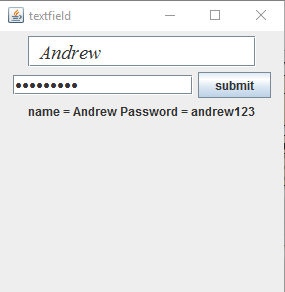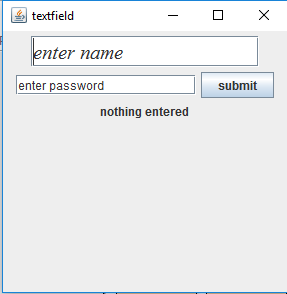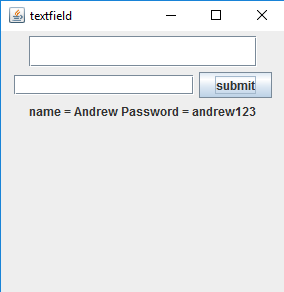PasswordField is a part of javax.swing package . The class JPasswordField is a component that allows editing of a single line of text where the view indicates that something was typed by does not show the actual characters. JPasswordField inherits the JTextField class in javax.swing package.

Constructors of the class are :

2. JPasswordField(int columns) : constructor that creates a new empty PasswordField with specified number of columns.
3. JPasswordField(String Password) : constructor that creates a new empty Password field initialized with the given string.
4. JPasswordField(String Password, int columns) : constructor that creates a new empty PasswordField with the given string and a specified number of columns .
5. JPasswordField(Document doc, String Password, int columns) : constructor that creates a Passwordfield that uses the given text storage model and the given number of columns.

Commonly used method of JPasswordField :

1. char getEchoChar() : returns the character used for echoing in JPasswordField.
2. setEchoChar(char c) : set the echo character for JPasswordField.
4. String getText() : returns the text contained in JPasswordField.

 `// Java Program to enter name and password ` `// using JTextField and JPasswordField ` `import` `java.awt.event.*; ` `import` `java.awt.*; ` `import` `javax.swing.*; ` `class` `text ``extends` `JFrame ``implements` `ActionListener { ` `    ``// JTextField ` `    ``static` `JTextField t; ` ` `  `    ``// JPasswodField ` `    ``static` `JPasswordField pass; ` ` `  `    ``// JFrame ` `    ``static` `JFrame f; ` ` `  `    ``// JButton ` `    ``static` `JButton b; ` ` `  `    ``// label to display text ` `    ``static` `JLabel l; ` ` `  `    ``// default constructor ` `    ``text() ` `    ``{ ` `    ``} ` ` `  `    ``// main class ` `    ``public` `static` `void` `main(String[] args) ` `    ``{ ` `        ``// create a new frame to store text field and button ` `        ``f = ``new` `JFrame(``"textfield"``); ` ` `  `        ``// create a label to display text ` `        ``l = ``new` `JLabel(``"nothing entered"``); ` ` `  `        ``// create a new button ` `        ``b = ``new` `JButton(``"submit"``); ` ` `  `        ``// create a object of the text class ` `        ``text te = ``new` `text(); ` ` `  `        ``// addActionListener to button ` `        ``b.addActionListener(te); ` ` `  `        ``// create a object of JTextField with 16 columns and initial text ` `        ``t = ``new` `JTextField(``"enter name"``, ``16``); ` ` `  `        ``// create a object of passwodField with 16 columns ` `        ``pass = ``new` `JPasswordField(``16``); ` ` `  `        ``// create an object of font type ` `        ``Font fo = ``new` `Font(``"Serif"``, Font.ITALIC, ``20``); ` ` `  `        ``// set the font of the textfield ` `        ``t.setFont(fo); ` ` `  `        ``// create a panel to add buttons and textfield ` `        ``JPanel p = ``new` `JPanel(); ` ` `  `        ``// add buttons and textfield to panel ` `        ``p.add(t); ` `        ``p.add(pass); ` `        ``p.add(b); ` `        ``p.add(l); ` ` `  `        ``// add panel to frame ` `        ``f.add(p); ` ` `  `        ``// set the size of frame ` `        ``f.setSize(``300``, ``300``); ` ` `  `        ``f.show(); ` `    ``} ` ` `  `    ``// if the button is pressed ` `    ``public` `void` `actionPerformed(ActionEvent e) ` `    ``{ ` `        ``String s = e.getActionCommand(); ` `        ``if` `(s.equals(``"submit"``)) { ` `            ``// set the text of the label to the text of the field ` `            ``l.setText(``"name = "` `+ t.getText() + ``"\t Password = "` `+ pass.getText()); ` ` `  `            ``// set the text of field to blank ` `            ``t.setText(``"  "``); ` ` `  `            ``// set the text of password field to blank ` `            ``pass.setText(``""``); ` `        ``} ` `    ``} ` `}`

output :2. Program to change the echo character of JPasswordField and set initial text for password field

 `// Java Program to change the echo character of ` `// JPasswordField and set initial text for password field ` `import` `java.awt.event.*; ` `import` `java.awt.*; ` `import` `javax.swing.*; ` `class` `text ``extends` `JFrame ``implements` `ActionListener, FocusListener { ` `    ``// JTextField ` `    ``static` `JTextField t; ` ` `  `    ``// JPasswodField ` `    ``static` `JPasswordField pass; ` ` `  `    ``// JFrame ` `    ``static` `JFrame f; ` ` `  `    ``// JButton ` `    ``static` `JButton b; ` ` `  `    ``// label to display text ` `    ``static` `JLabel l; ` ` `  `    ``// default constructor ` `    ``text() ` `    ``{ ` `    ``} ` ` `  `    ``// main class ` `    ``public` `static` `void` `main(String[] args) ` `    ``{ ` `        ``// create a new frame to store text field and button ` `        ``f = ``new` `JFrame(``"textfield"``); ` ` `  `        ``// create a label to display text ` `        ``l = ``new` `JLabel(``"nothing entered"``); ` ` `  `        ``// create a new button ` `        ``b = ``new` `JButton(``"submit"``); ` ` `  `        ``// create a object of the text class ` `        ``text te = ``new` `text(); ` ` `  `        ``// addActionListener to button ` `        ``b.addActionListener(te); ` ` `  `        ``// create a object of JTextField with 16 columns and initial text ` `        ``t = ``new` `JTextField(``"enter name"``, ``16``); ` ` `  `        ``// create a object of passwodField with 16 columns ` `        ``pass = ``new` `JPasswordField(``16``); ` ` `  `        ``// add FocusListener to passwordField ` `        ``pass.addFocusListener(te); ` ` `  `        ``// set the echo character of the password field ` `        ``pass.setEchoChar((``char``)``0``); ` ` `  `        ``// set initial text for password field ` `        ``pass.setText(``"enter password"``); ` ` `  `        ``// set the echo character of the password field ` `        ``// create an object of font type ` `        ``Font fo = ``new` `Font(``"Serif"``, Font.ITALIC, ``20``); ` ` `  `        ``// set the font of the textfield ` `        ``t.setFont(fo); ` ` `  `        ``// create a panel to add buttons and textfield ` `        ``JPanel p = ``new` `JPanel(); ` ` `  `        ``// add buttons and textfield to panel ` `        ``p.add(t); ` `        ``p.add(pass); ` `        ``p.add(b); ` `        ``p.add(l); ` ` `  `        ``// add panel to frame ` `        ``f.add(p); ` ` `  `        ``// set the size of frame ` `        ``f.setSize(``300``, ``300``); ` ` `  `        ``f.show(); ` `    ``} ` `    ``// flag to set the text to blank for the first time when the component gets focus ` `    ``boolean` `flag = ``true``; ` ` `  `    ``// events of focus listener ` `    ``// when focus is gained ` `    ``public` `void` `focusGained(FocusEvent e) ` `    ``{ ` `        ``if` `(flag) { ` `            ``// set a definite echo char ` `            ``pass.setEchoChar(``'*'``); ` ` `  `            ``// only set the text to blank for 1st time ` `            ``// set the text to blank ` `            ``pass.setText(``""``); ` `            ``flag = ``false``; ` `        ``} ` `    ``} ` ` `  `    ``// when the focus is lost ` `    ``public` `void` `focusLost(FocusEvent e) ` `    ``{ ` `    ``} ` ` `  `    ``// if the button is pressed ` `    ``public` `void` `actionPerformed(ActionEvent e) ` `    ``{ ` `        ``String s = e.getActionCommand(); ` `        ``if` `(s.equals(``"submit"``)) { ` `            ``// set the text of the label to the text of the field ` `            ``l.setText(``"name = "` `+ t.getText() + ``"\t, Password = "` `+ pass.getText()); ` ` `  `            ``// set the text of field to blank ` `            ``t.setText(``"  "``); ` ` `  `            ``// set the text of password field to blank ` `            ``pass.setText(``""``); ` `        ``} ` `    ``} ` `} `Note : The above programs might not run in an online compiler use an offline IDE.
the default text and number of columns of password can be changed by the programmer as per their need.

Attention reader! Don’t stop learning now. Get hold of all the important Java and Collections concepts with the Fundamentals of Java and Java Collections Course at a student-friendly price and become industry ready.

My Personal Notes arrow_drop_upThird year Department of Information Technology Jadavpur University

If you like GeeksforGeeks and would like to contribute, you can also write an article using contribute.geeksforgeeks.org or mail your article to contribute@geeksforgeeks.org. See your article appearing on the GeeksforGeeks main page and help other Geeks.

Please Improve this article if you find anything incorrect by clicking on the "Improve Article" button below.

Improved By : nidhi_biet, Akanksha_Rai

Article Tags :
Practice Tags :

Be the First to upvote.

Please write to us at contribute@geeksforgeeks.org to report any issue with the above content.